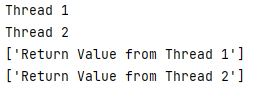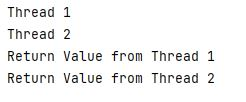# Get a Return Value From a Thread in Python

Dr. Muhammad Abdullah Sep 22, 2022

This article will first discuss thread basics and a code for starting a thread in Python. After this, we will discuss a code to get the value return from a function running in a thread.

A thread is a lightweight execution unit within a process with its own program execution states. A process runs multiple threads to achieve concurrency (and sometimes parallelism).

The main difference between a process and a thread is that each process has a separate disjoint address space, while multiple threads of the same process share the same address space of a single process. This means the threads can communicate using the shared memory without requiring additional pipes (Ordinary pipes or FIFOs) or any message passing interface.

## `HelloWorld` Program Using Multi-Threading in Python

Consider the following code:

``````from threading import Thread

def first_function():
print('Hello World')

print ("main program start")

print ("main ends")
``````

In the above code, first, we used the `from threading import Thread` statement to import the `Thread` class to use the multi-threading. We defined a function `first_function()` which displays `Hello World`, and used the `Thread()` class to instantiate the threads.

We created two instances of the `Thread()` class by passing the `first_function()` as a `target` function to run. The `target` attribute specifies the function to be executed by the `Thread()`.

Once the `Thread()` instances are created, we can run and execute these threads using the `.start()` method.

### Pass Arguments to a Function Running in Thread

Consider the following code:

``````from threading import Thread

def first_function(name, id):
print('Hello World from ', name, " ID= ", id)

``````

In the above code, we defined the function `first_function(name, id)`, receiving two arguments, `name` and `id`. We passed these arguments as a tuple using `args` in the `Thread` class.

We created two `Thread` class objects and passed the arguments `args=("Thread 1", 1)` and `args=("Thread 2", 2)` to `thread_1` and `thread_2` respectively. Further, `thread_1.start()` and `thread_2.start()` are used to run these threads.

## Get a Return Value From a Function Running in Thread in Python

There are different ways to get a return value from a function running in a thread.

### Pass a Mutable Object to the Function

We can get a value from a function running in a thread by passing a mutable object to the function; the function places the return value in that object. Consider the following code:

``````from threading import Thread

def first_function(first_argu, return_val):
print (first_argu)
return_val  = "Return Value from " + first_argu

return_val_from_1= [None]*1
return_val_from_2= [None]*1

print (return_val_from_1)
print (return_val_from_2)
``````

The above code defined a function `first_function` which receives two arguments: `first_argu` and `return_val`. The `first_function` display the value of `first_argu` and place the return value in the `0` indexes of `return_val`.

We create thread using `Thread` class and pass two arguments including a list ` args=("Thread 1", return_val_from_1)` and ` args=("Thread 2", return_val_from_2)` for `thread_1` and `thread_2` respectively. `return_val_from_1` and `return_val_from_2` are used to get value from the function.

`thread_1.join()` and `thread_2.join()` are used to wait for the main program to complete both threads.

Let’s look into the output for the above code snippet:### Use the `join` Method

The `join` method is another way to get a return value from a function running in a thread. Consider the following code:

``````from threading import Thread

def first_function(first_argu):
print (first_argu)
return "Return Value from " + first_argu

def __init__(self, group=None, target=None, name=None,
args=(), kwargs={}):
Thread.__init__(self, group, target, name, args, kwargs)
def run(self):
if self._target != None:
self._return = self._target(*self._args, **self._kwargs)
def join(self, *args):
return self._return

In the above code, we define a customized class, `NewThread`, a subclass of the `Thread` class. We redefine the `run` and `join` methods.
Once we create a thread and start, the return value from `first_function` is returned from the `join()` method.